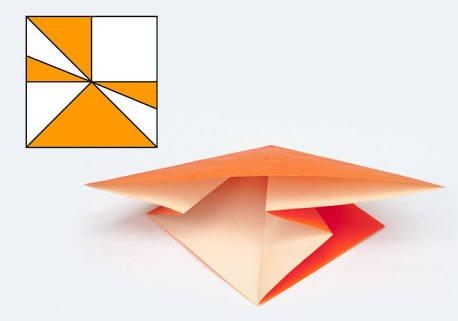# What Do You Know About Maekawa's Theorem?

10 Questions | Total Attempts: 106SettingsIn mathematics, Maekawa's theorem is a theorem used in the mathematics of paper folding. It basically involves flat-foldable origami crease patterns that are determined by a variation of valley-creases and mountain-creases. Maekawa's theorem states that the numbers of mountain folds and valleys always differ by two at every vertex—no matter the direction.

• 1.
Which aspect of mathematics is Maekawa theorem relevant.
• A.

Mathematics of setting cubes

• B.

Mathematics of folding paper

• C.

Mathematics of wave movement

• D.

Mathematics of scalar surfaces

• 2.
What does Maekawa's theorem propose that the total number of folds at each vertex must be?
• A.

An odd number

• B.

A decimal number

• C.

An even number

• D.

Half of the angle

• 3.
Who discovered this theorem before Maekawa worked on it?
• A.

S. Murata

• B.

B. Jurtala

• C.

Jan Nelson

• D.

Robert Oneil

• 4.
What is one major flaw of this theorem?
• A.

It does not completely characterize the flat-foldable vertices

• B.

It shows only the angles of the vertical cortex

• C.

It is only applicable to folds greater than 180 degrees

• D.

The theorem is mutually exclusive to angles of a straight shape

• 5.
When utilizing Maekawa's theorem, which one of the following is applicable at every vertex?
• A.

The number of mountain folds is the same in either direction

• B.

The number of valley and mountain folds is always the same in either direction

• C.

The numbers of valley and mountain folds always differ by two in either direction

• D.

The number of valley fold is the same in both directions of the vertex

• 6.
Which of the following is applicable when using Maekawa's theorem for a flat-foldable crease pattern?
• A.

It is not possible to color the regions of the creases

• B.

It is always possible to color the regions between the creases with two colors, such that each crease separates regions of differing colors

• C.

Each crease seperates the regions into 5 different colours

• D.

It is not possible to color the region creases with two colors but each crease can still be separated to regions of differing colors

• 7.
Which of these theorems is related to Maekawa's theorem?
• A.

Origami Theorem

• B.

Kawasaki Theorem

• C.

Murata Theorem

• D.

Vannelson Theorem

• 8.
Which of these scientists used the theorem with the same results in the same year it was discovered?
• A.

Jacques Justin

• B.

Hull Thomas

• C.

Takahama Inoshi

• D.

Craig Edwards

• 9.
In which mathematical publication was Maekawa's theorem first proposed?
• A.

Theorem of Wide Angles

• B.

Viva Origami

• C.

Theories of Origami Design

• D.

Mathematical Modeling Based on Origami Design

• 10.
Which mathematical problem prompted Jun Maekawa to design the Maekawa's theorem?
• A.

Angle wideness of origami models

• B.

Crease regions in origami models

• C.

Fold number in origami models

• D.

Flat-foldability of origami models

Related TopicsBack to top